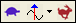Animation Speed

• Click on the turtle of the rabbiton the Toolbar to decrease or increase the animation speed

Data Block Sweep Speed

During sweep animation, the animation speed is controlled by how many samples of data are skipped or interpolated between

• For a speed of 1, every sample of data is displayed during a sweep

• For a speed of 2, every other sample is displayed, for a speed of 3, every third sample is displayed, and so on

• For a speed less than 1, shape values are calculated using linear interpolation between adjacent samples of data

• For a speed of 0.5, one interpolated value is calculated using half of the values at adjacent samples, for a speed of 0.33, two interpolated values are calculated, and so on.

Sine Dwell Speeds

During sine dwell animation, the animation speed is controlled by using a different number of sine values per cycle of animation.

• For a speed of 4, four sine values per cycle is used

• For a speed of (N>4), N sine values per cycle are used